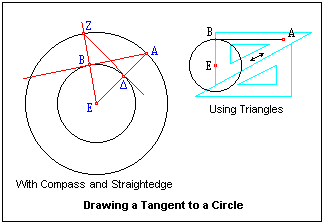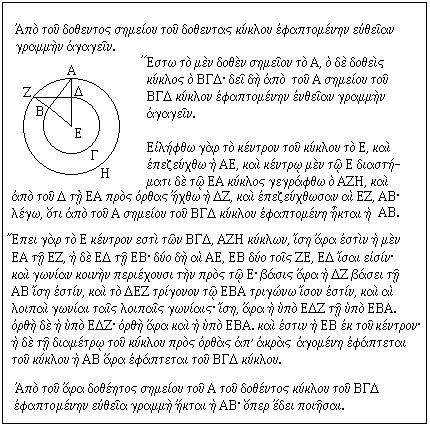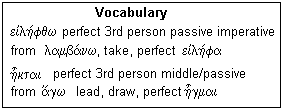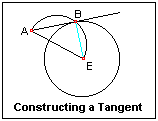## Lesson κβ': Drawing a Tangent to a Circle

This is one of the most common constructions in technical drawing

Book III, Proposition 17 is a problem of frequent application in technical drawing: From a given point, to draw a line tangent to a given circle. At this point, we know how to draw a line through two points (Postulate 1), a line through a point parallel to a given line (I-31), or perpendicular to a given line (I-12), or a perpendicular from a given point on a line (I-11). It seems that we must find the point of tangency in order to draw a line joining it and the given point. This is what is done in Euclid's solution of the problem.It is instructive to look at how this problem was solved when drawing by hand. Given the point and the circle, it is easy to draw the tangent by eye. This is not enough, however. The tangent point must also be found so that the point where the straight line ends and the circle begins is clear. This is necessary if the drawing is to be inked, for example. The tangent point is found by using the right angle of a triangle, as shown in the Figure. One edge of the triangle is brought parallel to the tangent that has just been drawn. A second triangle (or the T-square) is arranged so that the hypotenuse can slide along it, maintaining the direction. The triangle is then moved until the center of the circle, point E, is on the other leg. Now the point B where this side crosses the tangent can be marked.

Euclid's solution does not depend on a judgement of when a line is tangent to the circle, but finds the point of tangency B directly. Something of the sort is necessary for computer drawing as well, and the classical solutions can often be adapted, with the use of analytic geometry. In Euclid's solution, we first find the center E of the circle if necessary (III-1), then join AE. At the point Δ where this line intersects the circle, a perpendicular is drawn (I-11). A circle with radius EA is now drawn (Post. III). The perpendicular intersects the circle in Z. The line ZA then cuts the given circle at the point of tangency, B. To show that this works, we only have to prove that the angle EBA is a right angle. This is done by showing that the triangle ABE is congruent to triangle BΔE (SAS), and noting that angle EΔB is a right angle by construction.Here is the proposition in Greek. The statement is "From the given point draw a straight line touching the given circle." Then, "Let A be the given point, and BΓΔ the given circle. It is required to draw a straight line from the point A touching the circle BΓΔ. Take the center E of the circle and join AE, and with center E and distance EA draw the circle AZH, and from Δ ΔZ perpendicular to EA, and join EZ, AB; I say, that AB has been drawn from the point A touching the circle BΓΔ." Note that it has been necessary to change the word order greatly to make reasonable English.

Now the demonstration: "Since indeed E is the center of the circles BΓΔ, AZH, EA is equal to EZ on the one hand, and EΔ to EB on the other. The two AE, EB are indeed equal to the two ZE, EΔ, and [have] the common angle at E; therefore the base ΔZ it equal to the base AB, and the triangle ΔEZ is equal to the triangle EBA, and the other angles to the other angles [respectively]. Therefore, the [angle] EΔZ is equal to EBA. But EΔZ is a right angle; therefore, EBA is also right. Furthermore, EB is from the center; But the [line] drawn perpendicular to the diameter of a circle at its extremity touches the circle. AB, therefore, touches the circle BΓ&Delta." Now all that remains is to restate the proposition: "Therefore, from the given point A to the given circle BΓΔ the straight line AB has been drawn, which was to have been done."There are few unfamiliar words in the proposition. In the Vocabulary box, two verbs are reviewed whose forms used in the proposition do not resemble the first principal parts given in dictionaries. Both are used as passive perfects, one indicative, the other imperative. A/)gw is odd in that the γ is modified to κ at places in this tense for euphonic reasons. a/)kraj is the genitive of h( a/)kra, "promontory" or "summit", used here figuratively for the extremity of a diameter. The related adjective gives Acropolis, a citadel or fortress on a hill, the "high city."Another way to construct a tangent is shown in the Figure at the right. Draw AE from the given point A to the center of the circle. Then bisect this line and draw a circle with diameter AE. This circle cuts the given circle at the point of tangency B. The construction follows from the proposition that the angle in a semicircle is a right angle. This angle is ABE, which shows that the line AB is perpendicular to the radius at the point where it touches the circle, and, in fact, touches the circle indeed. Euclid does not use this proof since the proposition on which it depends, Proposition 31, comes later. This shows once again that there is more than one way to prove a proposition, and arranging them in logical order of dependence is a difficult task.